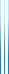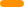# PublicationsPapers

## Agreement tests on graphs and hypergraphs

Irit Dinur, Yuval Filmus and Prahladh Harsha
Preliminary version in SODA'19

In the classical agreement test, we are given a black box which accepts a subset $S$ of $\{1,\ldots,n\}$ of size $k$, and outputs a binary vector of length $k$. The task is to determine whether the answers of the black box correspond to a global binary vector of length $n$. Dinur and Steurer showed that this property can be tested by picking two sets $S_1,S_2$ with intersection $k/2$, and comparing the answers of the black box on their intersection.

In this paper we generalize the results of Dinur and Steurer to the high-dimensional case, in which the black box outputs a bit for any $d$-tuple of coordinates, and the task is to determine whether the answers of the black box correspond to a 2-coloring of the complete $d$-uniform hypergraph on $n$ vertices. We show that for any constant $d$, the test proposed by Dinur and Steurer generalizes to this setting as well.

At the heart of our proof is a new hypergraph pruning lemma of independent interest. The lemma states that given a probability $p$, any $d$-uniform hypergraph $H$ can be pruned to a subhypergraph $H’$ such that $q_{=1}(H’)=\Omega(q_{>0}(G))$, where $q_{=1}(H’)$ is the probability that the subhypergraph of $H’$ induced by a random subset of the vertices chosen according to $\mu_p$ contains exactly one hyperedge, and $q_{>0}(H)$ is defined analogously. Crucially, the hidden constant depends on $d$ but not on $p$.

In the companion work Low degree almost Boolean functions are sparse juntas, we apply the new agreement test to prove a Kindler–Safra theorem for the biased Boolean cube. The merged version of both papers also contains another application: a generalization of the low degree test for the biased Boolean cube.

The conference version contains a mistake, which we have since corrected.

## BibTeX

@misc{DFH2020+,
author = {Irit Dinur and Yuval Filmus and Prahladh Harsha},
title = {Agreement tests on graphs and hypergraphs},
year = {2020+},
}

@inproceedings{DFH2019,
author = {Irit Dinur and Yuval Filmus and Prahladh Harsha},
title = {Analyzing boolean functions on the biased hypercube via higher-dimensional agreement tests},
booktitle = {ACM-SIAM Symposium on Discrete Algorithms (SODA19)},
year = {2019}
}copy to clipboard